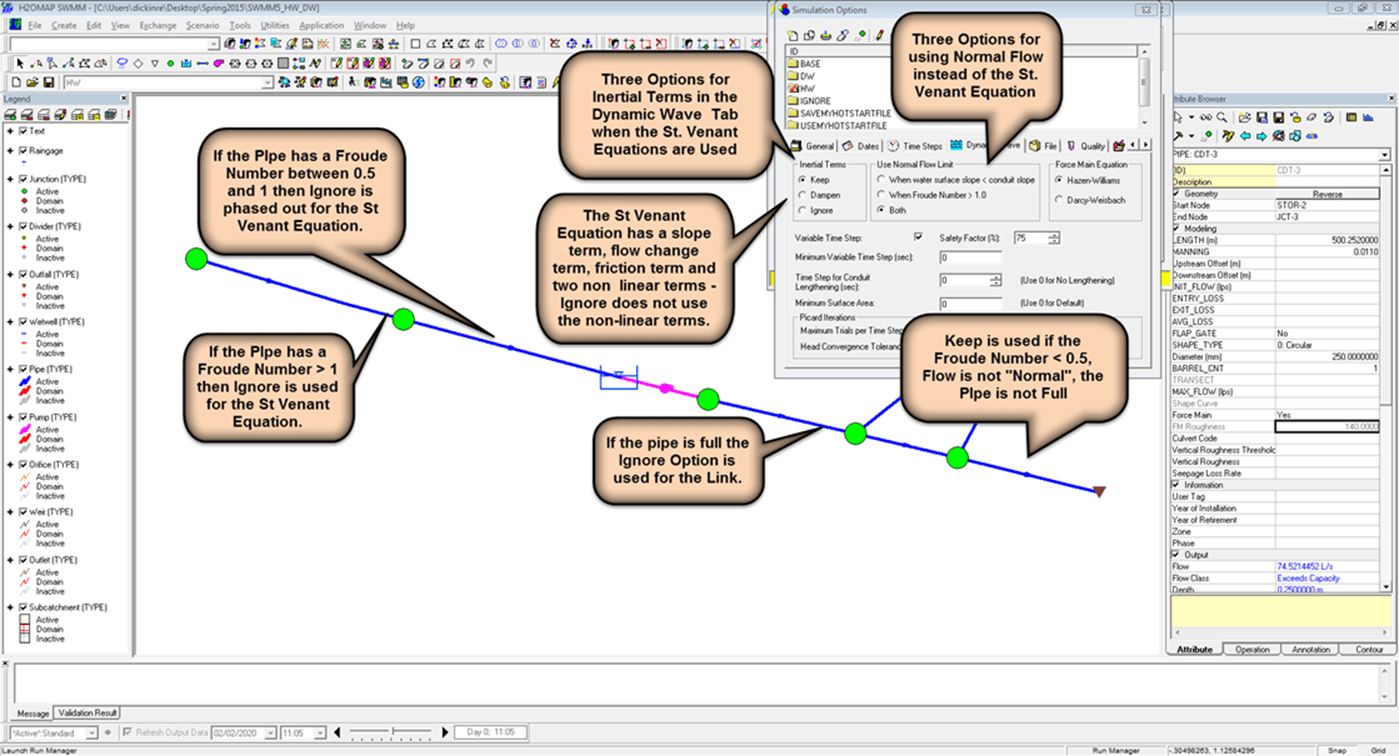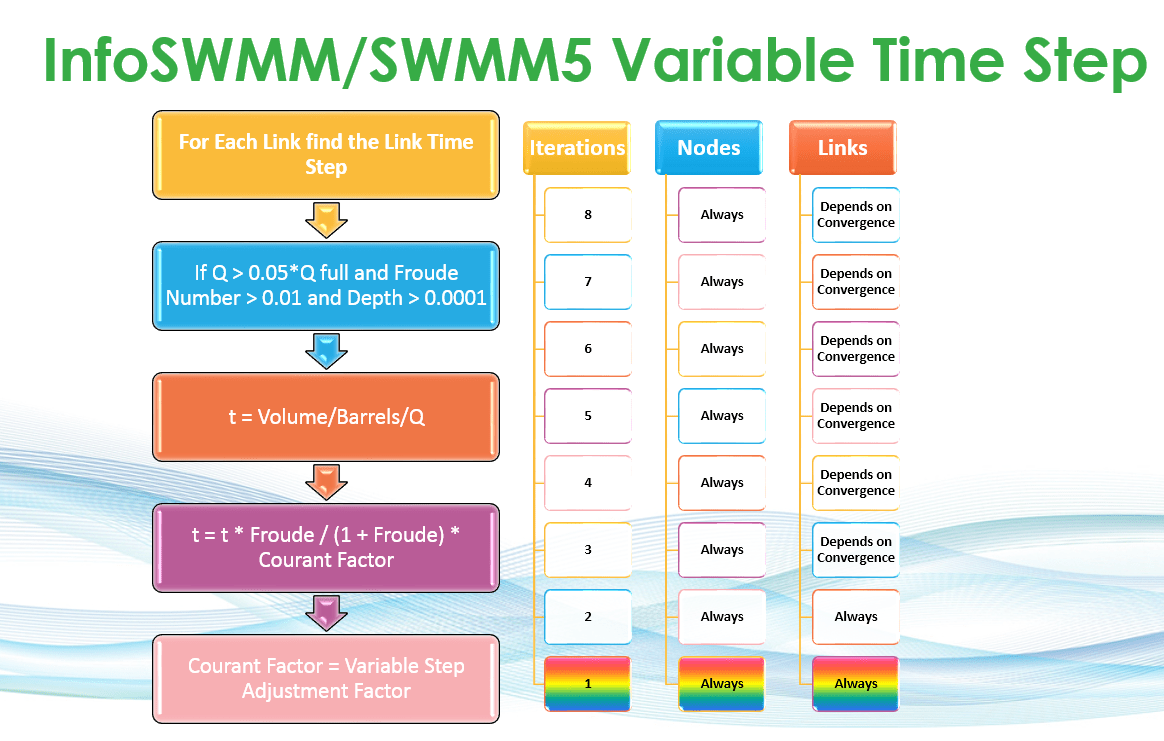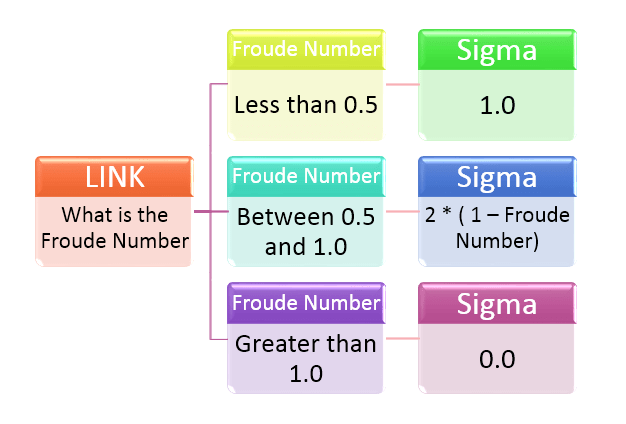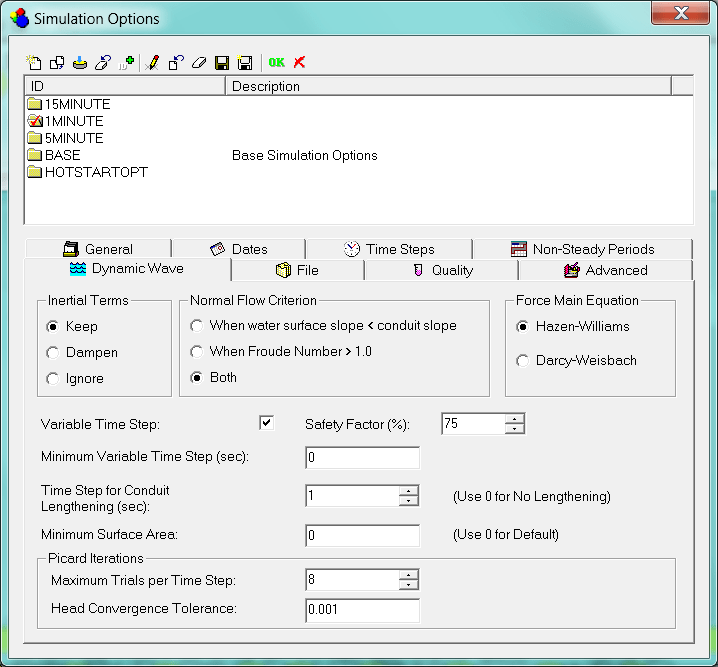#INFOSWMM

# Dynamic Wave Routing Options in InfoSWMM and InfoSWMM SA for the EPA SWMM 5 Engine

The Dynamic Wave page of the Simulation Options dialog, shown below, sets several parameters that control how the dynamic wave flow routing computations are made. These parameters have no effect for the other flow routing methods.Contents of this simulation option dialog page are described below:

 Name Description Inertial Terms Indicates how the inertial terms in the St. Venant momentum equation will be handled. KEEP maintains these terms at their full value under all conditions. Note: It may be helpful in some situations to try simulating the model with one of the other two options if you have a model with high continuity error or if you are trying to match output results from another hydraulic model (e.g. SWMM 4). DAMPEN reduces the terms as flow comes closer to being critical and ignores them when flow is supercritical. IGNORE drops the terms altogether from the momentum equation, producing what is essentially a Diffusion Wave solution.Variable Time Step Indicates whether or not a variable time step should be used. The variable step is computed for each time period so as to satisfy the Courant stability criterion for each conduit and to prevent an excessive change in water depth at each node. The Routing Time Step Summary Report is available for checking statistics about how InfoSWMM is altering the timestep. Safety Factor – This is a safety factor, between 10 and 200%, which is applied to the variable time step computed from the Courant criterion. It only applies when the Variable Time Step option is checked. The Safety Factor is multiplied times the time step to determine the actual time step used by the simulation. If this factor is too low the simulation may take longer than necessary and if too high the simulation may take longer to find an acceptable time step. By Default this factor is %100.Time Step for Conduit Lengthening This is a time step, in seconds, used to artificially lengthen conduits so that they meet the Courant stability criterion under full-flow conditions (i.e., the travel time of a wave will not be smaller than the specified conduit lengthening time step). As this value is decreased, fewer conduits will require lengthening. A value of 0 means that no conduits will be lengthened. The roughness value of the conduit will also be artificially lowered so that the same velocity and flow are maintained after lengthening. Also note that the conduit slope reported in the output will reflect a numerically smaller slope due to a longer conduit length. Conduit Lengthening in InfoSWMM H2OMap SWMM InfoSWMM SA If you use the conduit lengthening option in InfoSWMM H2OMap SWMM InfoSWMM SA then your short conduits will be lengthened based on the CFL or explicit time step criterion. Any conduits in which the Length Factor or the courant time step link length over the original length is greater than 1 will be lengthened and will have its roughness lowered so that the conduit is hydraulically the same at full conduit depth. The full area, full width and full hydraulic radius stay the same in the modified link – only the length, slope and roughness are altered. Length Factor = (Wave Celerity + Full Depth Velocity) * Time Step / Conduit Length, and for those links in which the Length Factor is greater than 1 New Roughness = Old Roughness / (Length Factor) ^1/2 New Slope = Old Slope / Length Factor A few metric’s for showing how this option has altered the network are shown in the figure below: 1. The most important is the increase in Network full volume as you never want to drastically alter the volume of your network, 2. The number of conduits modified along with the new mean slope (lower) and the new total conduit length are important indicators, 3. The mean wave celerity, full flow velocity and courant time step mean give the user some idea of the optimal time step for the simulation. Minimum Surface Area This is a minimum surface area used at nodes when computing changes in water depth. If 0 is entered, then the default value of 12.566 ft2 (i.e., the area of a 4-ft diameter manhole) is used.Use Normal Flow Limit Selects which condition is used to make InfoSWMM H2OMap SWMM InfoSWMM SA limit the flow in a conduit to the normal flow computed from the Manning equation: When water surface slope < conduit slope – the water surface slope is less than the conduit slope. When Froude Number > 1.0 – the Froude number at either end of the conduit is greater than 1.0 (i.e., the flow is supercritical). Both – Consider both Froude Number and slope information to check if supercritical condition has occurred. The effect of these three options is on the value of sigma for the non linear term in the St Venant Equation; a sigma value of 1 means that the whole term is used, a value of 0 means that the non linear term is not used for that time step.Force Main Equation Choose the head loss equation to be used to model force mains Maximum Number of Iterations Allows you to control the maximum number of iterations in the solution. The number of iterations range from 2 to a possible 20 iterations. Stopping Tolerance Controls the Stopping tolerance (internal units of feet) for node iterations.

Two new parameters and a modified table in the output starting in InfoSWMM 11 and H2OMAP SWMM v10 that does the following:

1. Allows you to control the maximum number of iterations in the solution,

2. Controls the Stopping tolerance (internal units of feet) for node iterations, and

3. Shows not only the percent continuity error at a node but the error in million gallons (Mgal)

If you have a high continuity error or want to reduce your existing continuity error then you can increase the number of iterations or lower the stopping tolerance so that at each time step there is less continuity error.